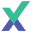# Discrete Fourier Transform in ExcelIn this video, we demonstrate the use of the Discrete Fourier Transform function in Excel to transform sample data into its frequency components and to re-construct it using the inverse DFT.

For our example, we'll use sample data simulated from ARMA(2,1) process.

## Script for Discrete Fourier Transform video

### Scene 1: Introduction

Welcome to the Discrete Fourier Transform (DFT) tutorial. In this video, we'll demonstrate the use of the DFT to transform sample data into its frequency components and to reconstruct it using the inverse DFT. For our example, we'll use sample data simulated from ARMA 2 1 process.

First, type in the DFT function in the formula bar for the F-18 cell, then click on the FX button on the right of the formula bar.

### Scene 2: DFT in Excel function dialog

The function argument dialog pops up. Select the cell range of the sample data. Hit F4 and lock the cell range. For the data chronicle order select one. For the component select the frequency component index or step reference the cell and E18. Lock the cell for column movement. For the return type select amplitude or type 1, now click OK.

### Scene 3:

The amplitude of 0 frequency component is shown. Copy the formula to the cell on the right, now click on the cell in G18 and click F2 to edit. Click on the FX button to the left of the formula bar.

### Scene 4:

In the function argument change the return type value to 2 for phase output.

### Scene 5:

The function returns 0 phase for zero frequency component. Next select the F18 cell and copy the formula to the rows below it. Select the G18 cell as well and copy the formula to the cells below it.

Now let's plot the spectral density amplitude on a bar diagram. Select the cell starting with the first component. Select a 2D column type diagram.

### Scene 6:

Next, let's reconstruct the time series using a subset of the frequency spectrum. Type IDFT in the formula bar and click on the FX button, IDFT stands for Inverse Discrete Fourier Transform.

### Scene 7:

The function argument dialog pops up. In the amplitude input select the cells range with amplitude values starting with component zero. Now lock the cell range. For the phase input select the cell range of the phase. The number of cells must match the amplitude cells range. For N enter the number of observations in the original sample data.

### Scene 8:

The IDFT function returns an array, but since we have one cell only the first cell is displayed. Select all the cells below it, hit F2, then press, ctrl, shift and enter together. The rest of the returned array is copied to the selected cells. Note the italic brackets around the formula, this indicates the array return type of the function.

Next, let's plot the new time series in the same graph as the original data.

### Scene 9:

The red curve is the filter time series or the one we constructed using a subset of the frequency spectrum of the original time series. Let's include more frequency components now. Select the cells in column C, then press F2. Resize the selected amplitude and phase cell range to include component six. Again press ctrl, shift and enter together to update the cell range.

### Scene 10:

The red curve is updated in the graph, it is following the original signal better. OK let's try to add more frequency components.

### Scene 11:

Resize the selected components all the way to 20, press ctrl, shift and enter to update the cells.

### Scene 12:

Now the red curve is updated and it is tracking the original signal very closely.

### Scene 13:

That is it for now, thank you for watching!

### Comments

Please sign in to leave a comment.

Was this article helpful?
3 out of 7 found this helpful